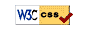# UM Math 116 On-Line Homework W21

## Course Selector

Read the hints and tips before taking a test. Select your section, and click "go to homework."

 Select Section Then Click section #
Note:
• Your username for the homework is your uniqname.
• You log in to the homework through the UM weblogin system. Thus, your password is your UM Kerberos password.
• Assignment due dates are shown in the homework.

## Hints and Tips

1. Start early, when each set opens.
2. Do the example homework set, Chap0 or homework0. It's easy & will help you with the homework system.
3. Don't submit partial answers. You have six submissions per problem.
4. Work the problems out by hand before entering them in the system. You can get a hardcopy of a problem set from the problem listing page of a problem set.
5. Preview your answers before submitting them.
6. Use calculator syntax:
1. Carefully parenthesize. Type `cos(2pi)`, `e^(-ln(3))`, or `1/(1+x)`, not `cos 2pi`, `e^-ln3` or `1/1+x`. Note that `sin2x` is read as `sin(2)*x`, which is probably not what you want. Enter `sin(2x)` instead.
2. The square root is `sqrt(x)` or `x^(1/2)`.
7. Watch your case: `X` is different from `x`, `Sin` is different from `sin`, and `Pi` is different from `pi`. You want `pi`.
8. Watch your precision. The system generally requires that numeric answers be correct at least four or five significant figures in an answer before it.UM Math 116 On-Line HW
Last modified: Wed Jan 21 10:05:00 EST 2021
Comments to: math-itc(at)umich(dot)edu
©2006-09 Gavin LaRose, UM Math Dept/Regents of UMich